# Queens on a donut

The eight queens problem is to place eight queens on a chessboard so that no queen attacks another. Because queens are allowed to move any number of spaces horizontally, vertically, or diagonally, this means no queen can be on the same row, column, or diagonal as any other queen.

For example, the following image gives one solution to the eight queens problem. (See the previous post for how I made the chessboard images in this post.)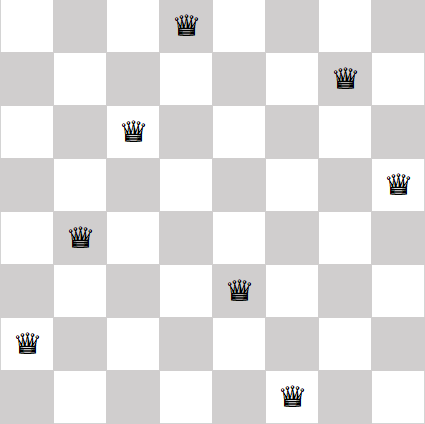The eight queens problem has been generalizes many ways, first by considering square chessboards of different sizes. The n-queens problem works on an n by n board. The n-queens problem can be solved for all n greater than 3.

The problem I want to consider here is the toroidal n queens problem. We first curl up our chessboard into a cylinder, then curl join the ends of the cylinder together to make a torus (donut).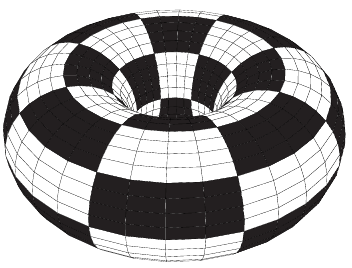(I found the image above on TeX StackExchange.)

When we do turn our chessboard into a torus, the rows and columns don’t change. If no two queens were on the same row (column) on the flat chessboard, there still won’t be any two queens on the same row (column) in the toroidal chessboard.

But the diagonals do change, as the following image illustrates.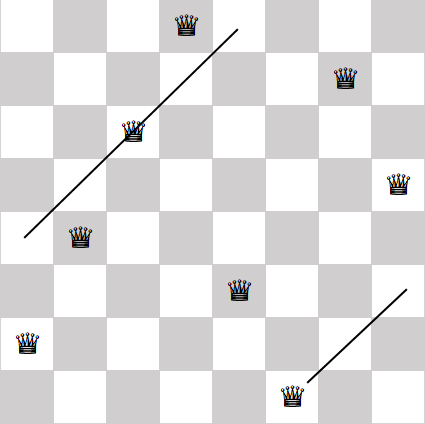If we curl our chessboard vertically by joining the left and right edges—no need to imagine the torus for this example, a cylinder will do—then some of the diagonals merge. The queen on the bottom row would be on the same diagonal as the queen in the third row from the top.

George Pólya proved that the toroidal n queens problem has a solution if and only if n is not a multiple of 2 or 3. Not only does the particular solution to the eight queens problem above not extent to a torus, no solution to the eight queens problem extends to a torus because 8 is divisible by 2.

The smallest non-trivial chessboard of size n with n not divisible by 2 or 3 is n = 5. The following solution to the five queens problem remains a solution if you identify the edges, making the board into a torus.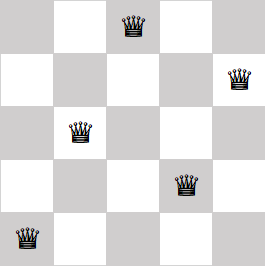# How to make a chessboard in Excel

I needed to make an image of a chessboard for the next blog post, and I’m not very good at image editing, so I make one using Excel.

There are Unicode characters for chess pieces— white king is U+2654, etc.—and so you can make a chessboard out of (Unicode) text.

`    ♔♕♖♗♘♙♚♛♜♝♞♟`

I placed the character for each piece in an cell and changed the formatting for all the cells to be centered horizontally and vertically. The following is a screenshot of the Excel file.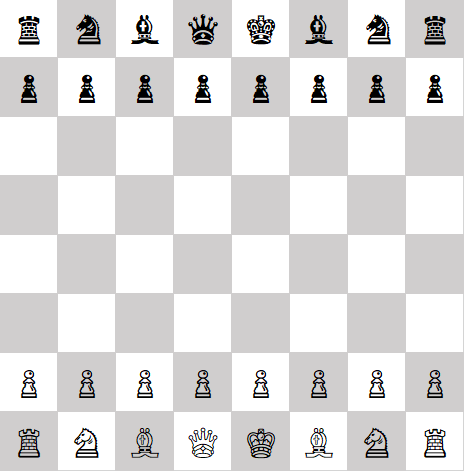The trickiest part is getting the cells to be square. By default Excel uses different units for height and width, with no apparent way to change the units. But if you switch the View to Page Layout, you can set row height and column width in inches or centimeters.

Another quirk is that you may have to experiment with the font to get all the pieces the same size. In some fonts, the black pawns were larger than everything else .

You can download my Excel file here. You could make any chessboard configuration with this file by copying and pasting characters where you want them.

When I tested the file in Libre Office, it worked, but I had to reset the row height to match the column width.

## Related posts

 Thanks to a reply on twitter I now understand why black’s pawn is sometimes outsized. The black pawn is used as an emoji, a sort of synecdoche representing chess. That’s why some fonts treat U+265E, black knight, entirely differently than U+265F, black pawn. The latter is interpreted not as a peer of the other pieces, but as the chess emoji. See the chess pawn entry in Emojipedia.

# King of high dimensional spaceWhich has more mobility on an empty chessboard: a rook, a king or a knight?

On an ordinary 8 by 8 chessboard, a rook can move to any one of 14 squares, no matter where it starts. A knight and a king both have 8 possible moves from the middle of the board, fewer moves from an edge.

If we make the board bigger or higher dimensional, what happens to the relative mobility of each piece? Assume we’re playing chess on a hypercube lattice, n points along each edge, in d dimensions. So standard chess corresponds to n = 8 and d = 2.

A rook can move to any square in a coordinate direction, so it can choose one of n-1 squares in each of d dimensions, for a total of (n-1)d possibilities.

A king can move a distance of 0, 1, or -1 in each coordinate. In d dimensions, this gives him 3d – 1 options. (Minus one for the position he’s initially on: moving 0 in every coordinate isn’t a move!)

What about a knight? There are C(d, 2) ways to choose two coordinate directions. For each pair of directions, there are 8 possibilities: two ways to choose which direction to move two steps in, and then whether to move + or – in each direction. So there are 4d(d – 1) possibilities.

In short:

• A king’s mobility increases exponentially with dimension and is independent of the size of the board.
• A rook’s mobility increases linearly with dimension and linearly with the size of the board.
• A knight’s mobility increases quadratically with dimension and independent of the size of the board.

The rules above are not the only way to generalize chess rules to higher dimensions. Here’s an interesting alternative way to define a knight’s moves: a knight can move to any lattice point a distance √5 away. In dimensions up to 4, this corresponds to the definition above. But in dimension 5 and higher, there’s a new possibility: moving one step in each of five dimensions. In that case, the number of possible knight moves increases with dimension like d5.

Related post: A knight’s random walk in 3 or 4 dimensions

# 3D chess knight moves

I’ve written before about a knight’s random walk on an ordinary chess board. In this post I’d like to look at the generalization to three dimensions (or more).So what do we mean by 3D chess? For this post, we’ll have a three dimensional lattice of possible positions, of size 8 by 8 by 8. You could think of this as a set of 8 ordinary chess boards stacked vertically. To generalize a knight’s move to this new situation, we’ll say that a knight move consists of moving 2 steps in one direction and 1 step in an orthogonal direction. For example, he might move up two levels and over one position horizontally.

Suppose our knight walks randomly through the chess lattice. At each point, he evaluates all possible moves and chooses one randomly with all possible moves having equal probability. How long on average will it take our knight to return to where he started?

As described in the post about the two dimensional problem, we can find the average return time using a theorem about Markov chains.

The solution is to view the problem as a random walk on a graph. The vertices of the graph are the squares of a chess board and the edges connect legal knight moves. The general solution for the time to first return is simply 2N/k where N is the number of edges in the graph, and k is the number of edges meeting at the starting point.

The problem reduces to counting N and k. This is tedious in two dimensions, and gets harder in higher dimensions. Rather than go through a combinatorial argument, I’ll show how to compute the result with a little Python code.

To count the number of edges N, we’ll add up the number of edges at each node in our graph, and then divide by 2 since this process will count each edge twice. We will iterate over our lattice, generate all potential moves, and discard those that would move outside the lattice.

```from numpy import all
from itertools import product, combinations

def legal(v):
return all([ 0 <= t < 8 for t in v])

count = 0
for position in product(range(8), range(8), range(8)):
# Choose two directions
for d in combinations(range(3), 2):
# Move 1 step in one direction
# and 2 steps in the other.
for step in [1, 2]:
for sign0 in [-1, 1]:
for sign1 in [-1, 1]:
move = list(position)
move[d] += sign0*step
move[d] += sign1*(3-step)
if legal(move):
count += 1
print(count // 2)
```

This tells us that there are N = 4,032 nodes in our graph of possible moves. The number of starting moves k depends on our starting point. For example, if we start at a corner, then we have 6 possibilities. In this case we should expect our knight to return to his starting point in an average of 2*4032/6 = 1,344 moves.

We can easily modify the code above. To look at different size lattices, we could change all the 8’s above. The function `legal` would need more work if the lattice was not the same size in each dimensions.

We could also look at four dimensional chess by adding one more range to the position loop and changing the combinations to come from `range(4)` rather than `range(3)`. In case you’re curious, in four dimensions, the graph capturing legal knight moves in an 8 by 8 by 8 by 8 lattice would have 64,512 edges. If our knight started in a corner, he’d have 12 possible starting moves, so we’d expect him to return to his starting position on average after 5,275 moves.

# Castles and quantum mechanics

How are castles and quantum mechanics related? One connection is rook polynomials.

The rook is the chess piece that looks like a castle, and used to be called a castle. It can move vertically or horizontally, any number of spaces.

A rook polynomial is a polynomial whose coefficients give the number of ways rooks can be arranged on a chess board without attacking each other. The coefficient of xk in the polynomial Rm,n(x) is the number of ways you can arrange k rooks on an m by n chessboard such that no two rooks are in the same row or column.

The rook polynomials are related to the Laguerre polynomials by

Rm,n(x) = n! xn Lnmn(-1/x)

where Lnk(x) is an “associated Laguerre polynomial.” These polynomials satisfy Laguerre’s differential equation

x y” + (n+1-x) y‘ + k y = 0,

an equation that comes up in numerous contexts in physics. In quantum mechanics, these polynomials arise in the solution of the Schrödinger equation for the hydrogen atom.

# Solutions to knight’s random walk

My previous post asked this question:

Start a knight at a corner square of an otherwise-empty chessboard. Move the knight at random by choosing uniformly from the legal knight-moves at each step. What is the mean number of moves until the knight returns to the starting square?

There is a mathematical solution that is a little arcane, but short and exact. You could also approach the problem using simulation, which is more accessible but not exact.

The mathematical solution is to view the problem as a random walk on a graph. The vertices of the graph are the squares of a chess board and the edges connect legal knight moves. The general solution for the time to first return is simply 2N/k where N is the number of edges in the graph, and k is the number of edges meeting at the starting point. Amazingly, the solution hardly depends on the structure of the graph at all. It only requires that the graph is connected. In our case N = 168 and k = 2.

For a full explanation of the math, see this online book, chapter 3, page 9. Start there and work your way backward until you understand the solution.

And now for simulation. The problem says to pick a legal knight’s move at random. The most direct approach would be to find the legal moves at a given point first, then choose one of those at random. The code below achieves the same end with a different approach. It first chooses a random move, and if that move is illegal (i.e. off the board) it throws that move away and tries again.  This will select a legal move with the right probability, though perhaps that’s not obvious. It’s what’s known as an accept-reject random generator.

```from random import randint

# Move a knight from (x, y) to a random new position
def new_position(x, y):

while True:
dx, dy = 1, 2

# it takes three bits to determine a random knight move:
# (1, 2) vs (2, 1), and the sign of each
r = randint(0, 7)
if r % 2:
dx, dy = dy, dx
if (r >> 1) % 2:
dx = -dx
if (r >> 2) % 2:
dy = -dy

newx, newy = x + dx, y + dy
# If the new position is on the board, take it.
# Otherwise try again.
if (newx >= 0 and newx < 8 and newy >= 0 and newy < 8):
return (newx, newy)

# Count the number of steps in one random tour
def random_tour():
x, y = x0, y0 = 0, 0
count = 0
while True:
x, y = new_position(x, y)
count += 1
if x == x0 and y == y0:
return count

# Average the length of many random tours
sum = 0
num_reps = 100000
for i in xrange(num_reps):
sum += random_tour()
print sum / float(num_reps)
```

A theorem is better than a simulation, but a simulation is a lot better than nothing. This problem illustrates how sometimes we think we need to simulate when we don’t. On the other hand, when you have a simulation and a theorem, you have more confidence in your solution because each validates the other.

# A knight’s random walk

Here’s a puzzle I ran across today:

Start a knight at a corner square of an otherwise-empty chessboard. Move the knight at random by choosing uniformly from the legal knight-moves at each step. What is the mean number of moves until the knight returns to the starting square?

There’s a slick mathematical solution that I’ll give later.

You could also find the answer via simulation: write a program to carry out a knight random walk and count how many steps it takes. Repeat this many times and average your counts.Related post: A knight’s tour magic square

# A magic king’s tour

After posting about a magic square made from knight’s tour, I wondered whether there are magic squares made from a king’s tour. (A king can move one square in any direction. A tour is a sequence of moves that lands on each square of a chess board exactly once.) I found George Jelliss’ site via the comments to that post and found out that there are indeed magic king’s tours. Here’s one published in 1917.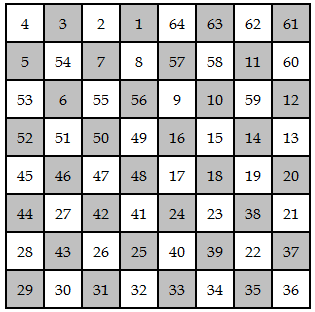Here’s the path a king would take in the square above:The knight’s tour magic square had rows and columns that sum to 260, though the diagonals did not. In fact, someone has proved that a knight’s tour on an 8×8 board cannot be diagonally magic. (Thanks John V.)

In the king’s tour above, however, the rows, columns, and diagonals all sum to 260. George Jelliss has posted notes that classify all such magic squares that have biaxial symmetry. See his site for much more information.

# A knight’s tour magic squareThis magic square was created by Leonhard Euler (1707-1783). Each row and each column sum to 260. Each half-row and half-column sum to 130. The square is also a knight’s tour: a knight could visit each square on a chessboard exactly once by following the numbers in sequence.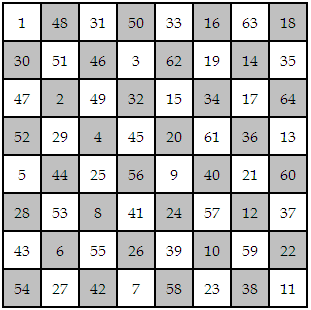Here is Python code to verify that the square has the properties listed above.

Update: It seems the attribution to Euler is a persistent error. Euler did publish the first paper on knight’s tours, but the knight’s tour square above was published by William Beverley in 1848. Thanks to George Jelliss for the correction. See the comments below.

Update 2: Notes from George Jelliss on magic king and queen tours.

Update 3: This is technically a semi-magic square: the rows add up to the same magic constant, but the diagonals do not. See Magic square errata.